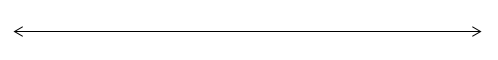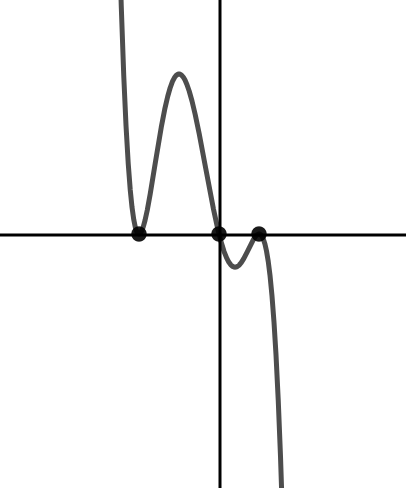# Polynomials

## Objective

Sketch polynomial functions using sign charts and analysis of the factored form of the polynomial function.

## Common Core Standards

### Core Standards

?

• A.APR.B.3 — Identify zeros of polynomials when suitable factorizations are available, and use the zeros to construct a rough graph of the function defined by the polynomial.

?

• F.IF.B.4

## Criteria for Success

?

1. Given the equation of a polynomial equation in factored form, identify whether the function is positive or negative between the roots.
2. Identify the general shape of the function based on the factors of the polynomial written in factored form.
3. Sketch polynomial functions by analyzing the interval between roots.
4. Write possible polynomial equations based on a sketch of a polynomial function and/or information about a particular polynomial function.

## Tips for Teachers

?

Brainstorming: Graphing a Polynomial Using a Sign Chart may be helpful to provide background knowledge on how to develop a sign chart for graphing functions.

## Anchor Problems

?

### Problem 1

Sketch a polynomial function with the following marked roots and that meets the conditions below.

• Positive when ${x<-3}$
• Negative when ${-3<x<0 }$
• Positive when ${ 0<x<2}$
• Positive when ${x>2}$### Problem 2

Below is a polynomial in factored form.

${y=-(x+1)(x+2)^2(3x)^2}$

Chart the roots on the number line below, and identify whether the function is positive or negative at the ends and between each root.## Problem Set

?

The following resources include problems and activities aligned to the objective of the lesson that can be used to create your own problem set.

• Include problems where students create a polynomial function based on constraints provided.
• Include problems where an incomplete set of constraints is given and students need to modify the constraints to match a given equation.
• Include an open graph of a polynomial for which students need to identify a possible equation based on constraints.

Below is a polynomial function with roots at ${x=1}$, ${x=-2}$,and ${x=0}$